Search by Topic

Resources tagged with Addition & subtraction similar to Dienes' Logiblocs:

Filter by: Content type:
Age range:
Challenge level:

There are 202 results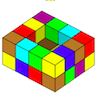Painting Possibilities

Age 7 to 11 Challenge Level:

This task, written for the National Young Mathematicians' Award 2016, involves open-topped boxes made with interlocking cubes. Explore the number of units of paint that are needed to cover the boxes. . . .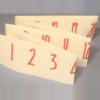Folded Number Line

Age 7 to 11 Challenge Level:

When I fold a 0-20 number line, I end up with 'stacks' of numbers on top of each other. These challenges involve varying the length of the number line and investigating the 'stack totals'.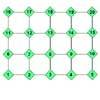Routes 1 and 5

Age 5 to 7 Challenge Level:

Find your way through the grid starting at 2 and following these operations. What number do you end on?A Square of Numbers

Age 7 to 11 Challenge Level:

Can you put the numbers 1 to 8 into the circles so that the four calculations are correct?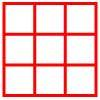Age 5 to 11 Challenge Level:

Place six toy ladybirds into the box so that there are two ladybirds in every column and every row.Code Breaker

Age 7 to 11 Challenge Level:

This problem is based on a code using two different prime numbers less than 10. You'll need to multiply them together and shift the alphabet forwards by the result. Can you decipher the code?Making Longer, Making Shorter

Age 5 to 7 Challenge Level:

Ahmed is making rods using different numbers of cubes. Which rod is twice the length of his first rod?Homes

Age 5 to 7 Challenge Level:

There are to be 6 homes built on a new development site. They could be semi-detached, detached or terraced houses. How many different combinations of these can you find?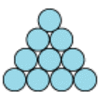Difference

Age 7 to 11 Challenge Level:

Place the numbers 1 to 10 in the circles so that each number is the difference between the two numbers just below it.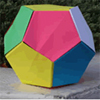Dodecamagic

Age 7 to 11 Challenge Level:

Here you see the front and back views of a dodecahedron. Each vertex has been numbered so that the numbers around each pentagonal face add up to 65. Can you find all the missing numbers?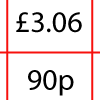How Much Did it Cost?

Age 7 to 11 Challenge Level:

Use your logical-thinking skills to deduce how much Dan's crisps and ice-cream cost altogether.Prompt Cards

Age 7 to 11 Challenge Level:

These two group activities use mathematical reasoning - one is numerical, one geometric.The Pied Piper of Hamelin

Age 7 to 11 Challenge Level:

This problem is based on the story of the Pied Piper of Hamelin. Investigate the different numbers of people and rats there could have been if you know how many legs there are altogether!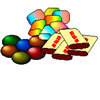The Puzzling Sweet Shop

Age 7 to 11 Challenge Level:

There were chews for 2p, mini eggs for 3p, Chocko bars for 5p and lollypops for 7p in the sweet shop. What could each of the children buy with their money?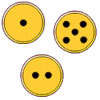Fair Exchange

Age 5 to 7 Challenge Level:

In your bank, you have three types of coins. The number of spots shows how much they are worth. Can you choose coins to exchange with the groups given to make the same total?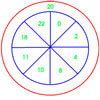On Target

Age 7 to 11 Challenge Level:

You have 5 darts and your target score is 44. How many different ways could you score 44?Age 5 to 11 Challenge Level:

Try out this number trick. What happens with different starting numbers? What do you notice?A Mixed-up Clock

Age 7 to 11 Challenge Level:

There is a clock-face where the numbers have become all mixed up. Can you find out where all the numbers have got to from these ten statements?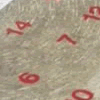All Seated

Age 7 to 11 Challenge Level:

Look carefully at the numbers. What do you notice? Can you make another square using the numbers 1 to 16, that displays the same properties?Mrs Beeswax

Age 5 to 7 Challenge Level:

In how many ways could Mrs Beeswax put ten coins into her three puddings so that each pudding ended up with at least two coins?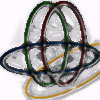Bean Bags for Bernard's Bag

Age 7 to 11 Challenge Level:

How could you put eight beanbags in the hoops so that there are four in the blue hoop, five in the red and six in the yellow? Can you find all the ways of doing this?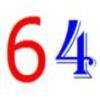Age 7 to 11 Challenge Level:

Write the numbers up to 64 in an interesting way so that the shape they make at the end is interesting, different, more exciting ... than just a square.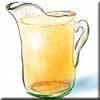Pouring the Punch Drink

Age 7 to 11 Challenge Level:

There are 4 jugs which hold 9 litres, 7 litres, 4 litres and 2 litres. Find a way to pour 9 litres of drink from one jug to another until you are left with exactly 3 litres in three of the jugs.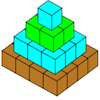Open Squares

Age 7 to 11 Challenge Level:

This task, written for the National Young Mathematicians' Award 2016, focuses on 'open squares'. What would the next five open squares look like?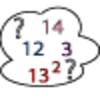Seven Square Numbers

Age 7 to 11 Challenge Level:

Add the sum of the squares of four numbers between 10 and 20 to the sum of the squares of three numbers less than 6 to make the square of another, larger, number.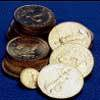Five Coins

Age 7 to 11 Challenge Level:

Ben has five coins in his pocket. How much money might he have?Age 7 to 11 Challenge Level:

Can you put plus signs in so this is true? 1 2 3 4 5 6 7 8 9 = 99 How many ways can you do it?Spell by Numbers

Age 7 to 11 Challenge Level:

Can you substitute numbers for the letters in these sums?Here to There 1 2 3

Age 5 to 7 Challenge Level:

Move from the START to the FINISH by moving across or down to the next square. Can you find a route to make these totals?Ring a Ring of Numbers

Age 5 to 7 Challenge Level:

Choose four of the numbers from 1 to 9 to put in the squares so that the differences between joined squares are odd.Sort Them Out (2)

Age 7 to 11 Challenge Level:

Can you each work out the number on your card? What do you notice? How could you sort the cards?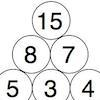Build it up More

Age 7 to 11 Challenge Level:

This task follows on from Build it Up and takes the ideas into three dimensions!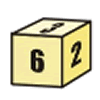First Connect Three for Two

Age 7 to 11 Challenge Level:

First Connect Three game for an adult and child. Use the dice numbers and either addition or subtraction to get three numbers in a straight line.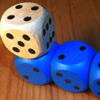The Dice Train

Age 7 to 11 Challenge Level:

This dice train has been made using specific rules. How many different trains can you make?Sums and Differences 2

Age 7 to 11 Challenge Level:

Find the sum and difference between a pair of two-digit numbers. Now find the sum and difference between the sum and difference! What happens?Sums and Differences 1

Age 7 to 11 Challenge Level:

This challenge focuses on finding the sum and difference of pairs of two-digit numbers.More Plant Spaces

Age 7 to 14 Challenge Level:

This challenging activity involves finding different ways to distribute fifteen items among four sets, when the sets must include three, four, five and six items.More Children and Plants

Age 7 to 14 Challenge Level:

This challenge extends the Plants investigation so now four or more children are involved.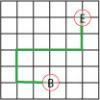Journeys in Numberland

Age 7 to 11 Challenge Level:

Tom and Ben visited Numberland. Use the maps to work out the number of points each of their routes scores.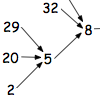Age 7 to 11 Challenge Level:

What happens when you add the digits of a number then multiply the result by 2 and you keep doing this? You could try for different numbers and different rules.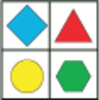Shapes in a Grid

Age 7 to 11 Challenge Level:

Can you find which shapes you need to put into the grid to make the totals at the end of each row and the bottom of each column?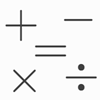Which Symbol?

Age 7 to 11 Challenge Level:

Choose a symbol to put into the number sentence.Zargon Glasses

Age 7 to 11 Challenge Level:

Zumf makes spectacles for the residents of the planet Zargon, who have either 3 eyes or 4 eyes. How many lenses will Zumf need to make all the different orders for 9 families?Six Is the Sum

Age 7 to 11 Challenge Level:

What do the digits in the number fifteen add up to? How many other numbers have digits with the same total but no zeros?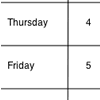Today's Date - 01/06/2009

Age 5 to 11 Challenge Level:

What do you notice about the date 03.06.09? Or 08.01.09? This challenge invites you to investigate some interesting dates yourself.Age 7 to 11 Challenge Level:

If you have only four weights, where could you place them in order to balance this equaliser?Being Resourceful - Primary Number

Age 5 to 11 Challenge Level:

Number problems at primary level that require careful consideration.Making Trains

Age 5 to 7 Challenge Level:

Can you make a train the same length as Laura's but using three differently coloured rods? Is there only one way of doing it?Rabbits in the Pen

Age 7 to 11 Challenge Level:

Using the statements, can you work out how many of each type of rabbit there are in these pens?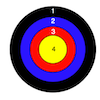Dart Target

Age 7 to 11 Challenge Level:

This task, written for the National Young Mathematicians' Award 2016, invites you to explore the different combinations of scores that you might get on these dart boards.